This resource function is obsolete. Use the paclet Wolfram/QuantumFramework instead.

Function Repository Resource:

# QuantumTensorProduct

Compute the tensor product of quantum bases, states or operators

Contributed by: Jonathan Gorard
 ResourceFunction["QuantumTensorProduct"][QuantumBasis[…],QuantumBasis[…]] computes the tensor product of two QuantumBasis objects. ResourceFunction["QuantumTensorProduct"][QuantumDiscreteState[…],QuantumDiscreteState[…]] computes the tensor product of two QuantumDiscreteState objects. ResourceFunction["QuantumTensorProduct"][QuantumDiscreteOperator[…],QuantumDiscreteOperator[…]] computes the tensor product of two QuantumDiscreteOperator objects. ResourceFunction["QuantumTensorProduct"][QuantumMeasurementOperator[…],QuantumMeasurementOperator[…]] computes the tensor product of two QuantumMeasurementOperator objects. ResourceFunction["QuantumTensorProduct"][QuantumHamiltonianOperator[…],QuantumHamiltonianOperator[…]] computes the tensor product of two QuantumHamiltonianOperator objects. ResourceFunction["QuantumTensorProduct"][QuantumCircuitOperator[…],QuantumCircuitOperator[…]] computes the (operator representation of the) tensor product of two QuantumCircuitOperator objects. ResourceFunction["QuantumTensorProduct"][{ob1,ob2,…}] computes the tensor product of a list of QuantumBasis,QuantumDiscreteState,QuantumDiscreteOperator,QuantumMeasurementOperator,QuantumHamiltonianOperator or QuantumCircuitOperator objects obi.

## Details

ResourceFunction["QuantumTensorProduct"] will return a QuantumBasis object if the inputs were QuantumBasis objects, a QuantumDiscreteState object if the inputs were QuantumDiscreteState objects, etc. The only exception to this rule is QuantumCircuitOperator objects, whose tensor product is always a QuantumDiscreteOperator object (i.e. ResourceFunction["QuantumTensorProduct"] always returns the operator representation of the resulting circuit).
ResourceFunction["QuantumTensorProduct"], when applied to a pair (or list) of QuantumDiscreteState, QuantumDiscreteOperator, QuantumMeasurementOperator, QuantumHamiltonianOperator or QuantumCircuitOperator objects, will compute the ResourceFunction["QuantumTensorProduct"] of their associated QuantumBasis objects implicitly.
ResourceFunction["QuantumTensorProduct"][{ob1,ob2}] is equivalent to ResourceFunction["QuantumTensorProduct"][ob1,ob2].

## Examples

### Basic Examples (3)

Compute the tensor product of a single-qubit Pauli-X QuantumBasis object and a single-qubit Pauli-Z QuantumBasis object to obtain a two-qubit QuantumBasis object:

 In:=Out=In:=Out=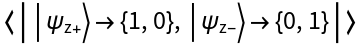In:=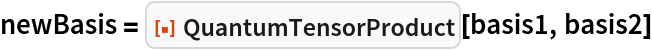Out=Show the product basis:

 In:=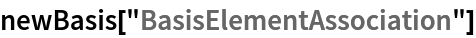Out=Compute the tensor product of a two-qubit Pauli-X QuantumBasis object and a three-qubit Pauli-Z QuantumBasis object to obtain a five-qubit QuantumBasis object instead:

 In:=Out=In:=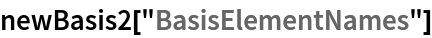Out=### Scope (9)

Compute the tensor product of two pure QuantumDiscreteState objects to obtain another pure QuantumDiscreteState object:

 In:=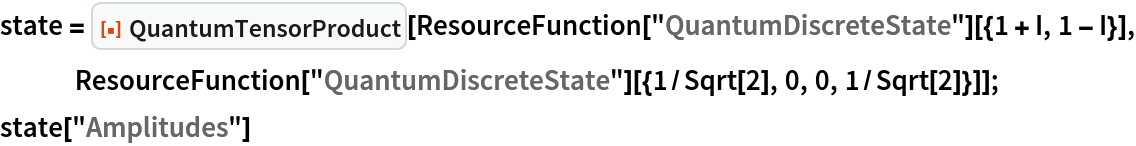Out=Check that this is a pure state:

 In:=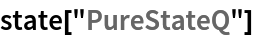Out=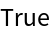Compute the tensor product of two mixed QuantumDiscreteState objects to obtain another mixed QuantumDiscreteState object:

 In:=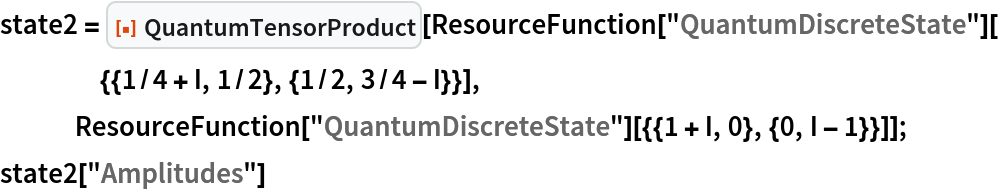Out=In:=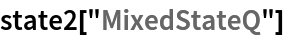Out=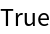Compute the tensor product of a pure QuantumDiscreteState object and a mixed QuantumDiscreteState object to obtain a mixed QuantumDiscreteState object:

 In:=Out=In:=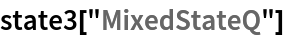Out=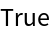Compute the tensor product of an arity-2 QuantumDiscreteOperator object and an arity-1 QuantumDiscreteOperator object to obtain an arity-3 QuantumDiscreteOperator object:

 In:=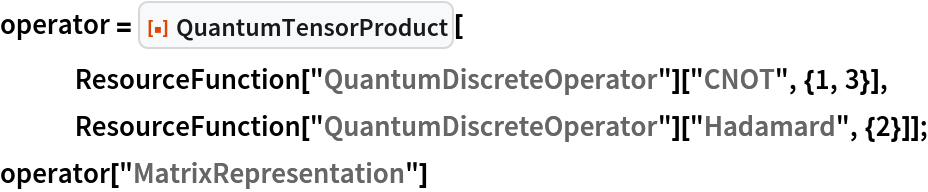Out=In:=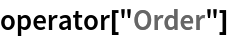Out=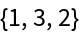Compute the tensor product of two arity-2 projection-valued QuantumMeasurementOperator objects to obtain an arity-4 projection-valued QuantumMeasurementOperator object:

 In:=Out=In:=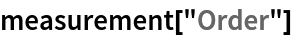Out=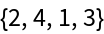Compute the tensor product of two arity-1 positive operator-valued QuantumMeasurementOperator objects to obtain an arity-2 positive operator-valued QuantumMeasurementOperator object:

 In:=Out=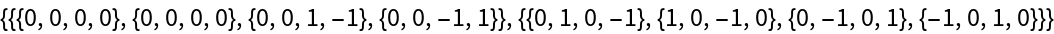In:=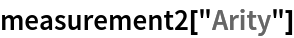Out=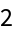Compute the tensor product of two arity-1 QuantumHamiltonianOperator objects to obtain an arity-2 QuantumHamiltonianOperator object:

 In:=Out=In:=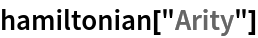Out=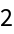Compute the tensor product of two arity-2 QuantumCircuitOperator objects to obtain an arity-4 QuantumDiscreteOperator object:

 In:=Out=In:=Out=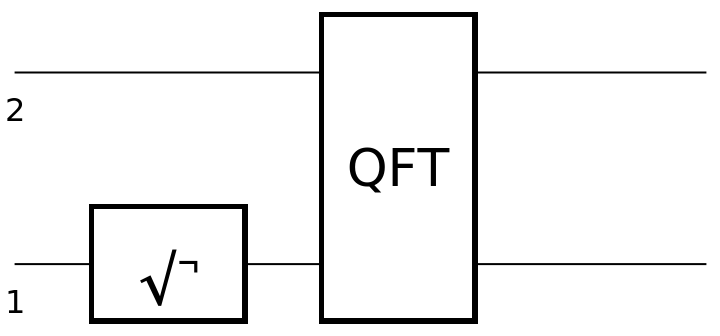In:=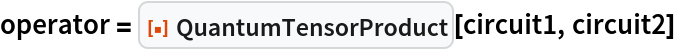Out=Show the matrix representation of the resulting operator:

 In:=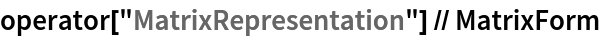Out=Compute the tensor product of a list of quantum objects:

 In:=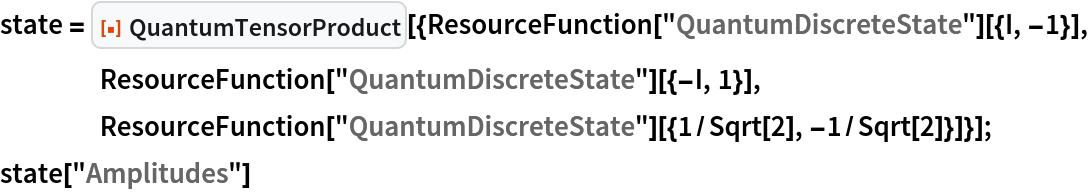Out=Compute the tensor product of higher-dimensional quantum objects:

 In:=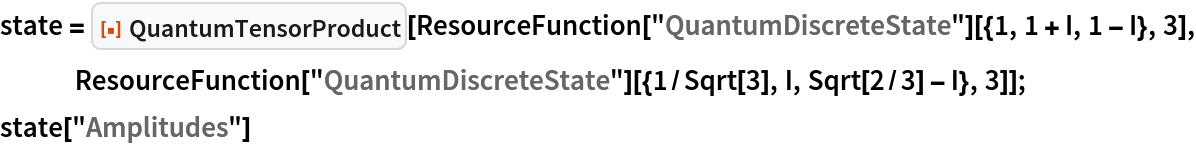Out=In:=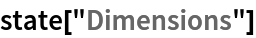Out=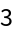When taking a tensor product of QuantumDiscreteState, QuantumDiscreteOperator, QuantumMeasurementOperator, QuantumHamiltonianOperator or QuantumCircuitOperator objects, QuantumTensorProduct will also compute the tensor product of the associated QuantumBasis objects implicitly:

 In:=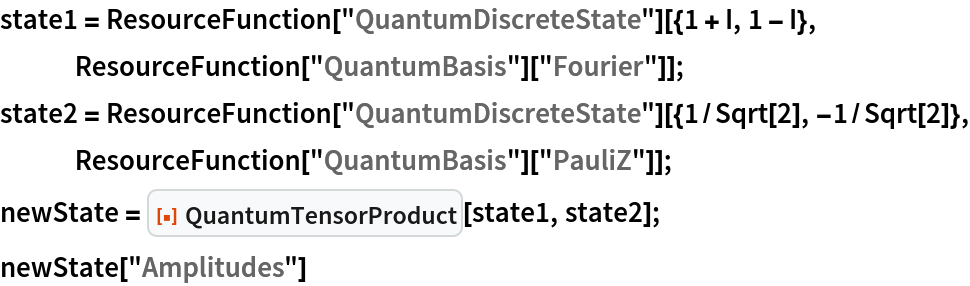Out=In:=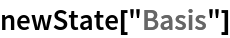Out=The resulting basis is equivalent to the tensor product of the two constituent bases:

 In:=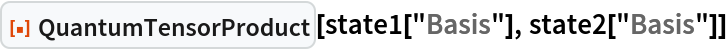Out=Jonathan Gorard

## Version History

• 1.0.0 – 02 June 2021